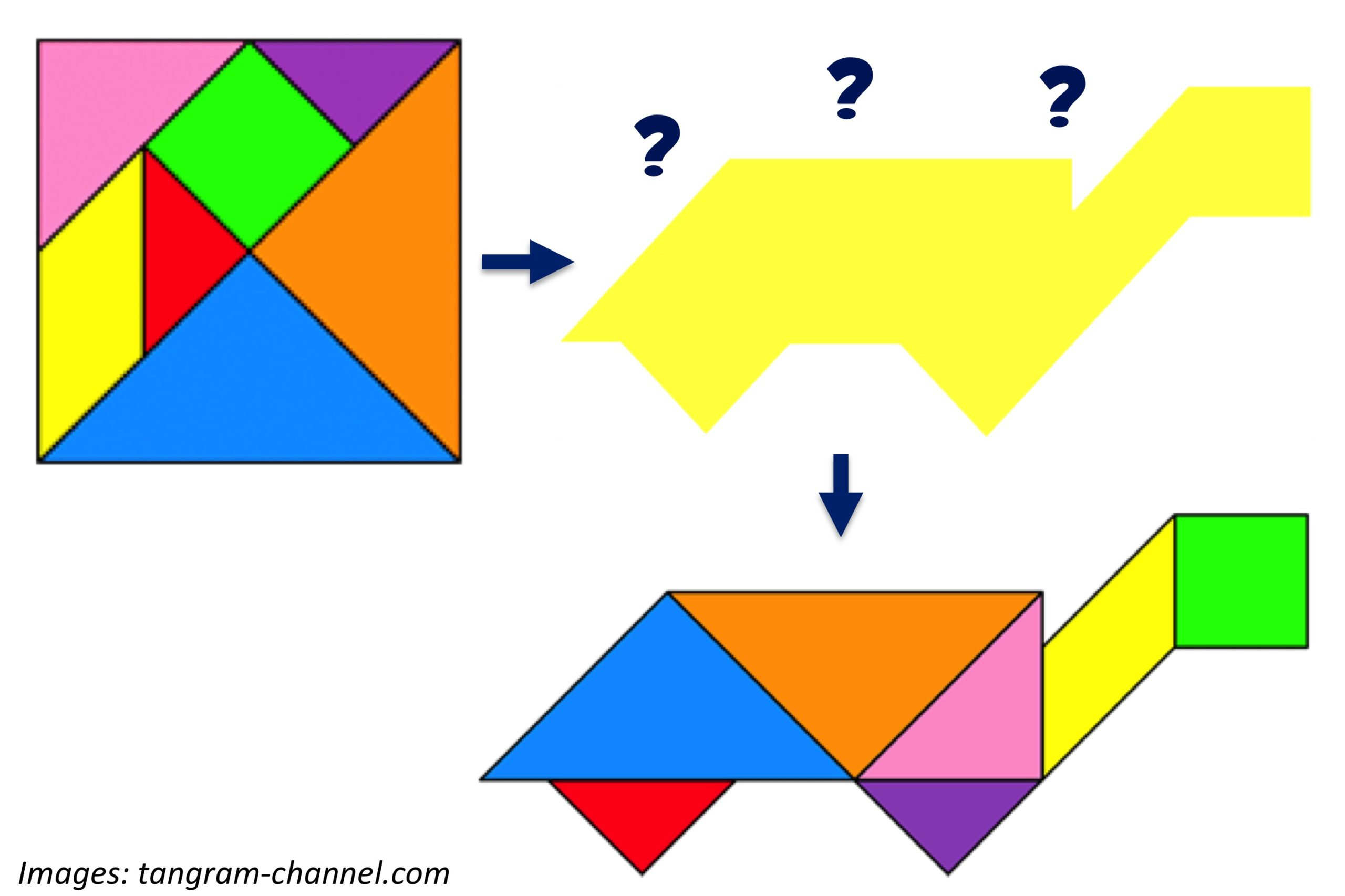# Tricky TrianglesThe word “puzzle” can mean a lot of things: peg puzzles, number games, and jigsaws with squiggly pieces. But our favorite at Bedtime Math is the “tangram.” This ancient Chinese puzzle uses 7 shapes that you move around to match a picture. The picture gives no clues about where the shapes go — you have to figure that out! Here we’ve made this turtle by lining up the shapes as shown. The 7 shapes fit with each other in a special way: the medium triangle is the same size as 2 tiny triangles put together, and a big triangle is the same as 2 medium ones. The square and parallelogram also each equal 2 tiny triangles. Check out this page to print and cut out your own tangrams, and to match some puzzles yourself!

Wee ones: Point to all the triangles in the picture. What colors are they?

Little kids: If 2 tiny triangles make a medium triangle, and 2 medium triangles make a big triangle, how many tiny triangles do you need to make a big triangle?  Bonus: The 2 tiny triangles against the green square make a shape called a “trapezoid.” Imagine that shape is all one color. How many sides does it have?

Big kids: How many tiny triangles can you fit in the whole big square that holds all the shapes? (Hint: the whole big square can fit 4 big triangles.) Bonus: If a giant tangram square covers 128 square inches, how much area does each tiny triangle cover? Can you figure out a handy way to divide by that number?